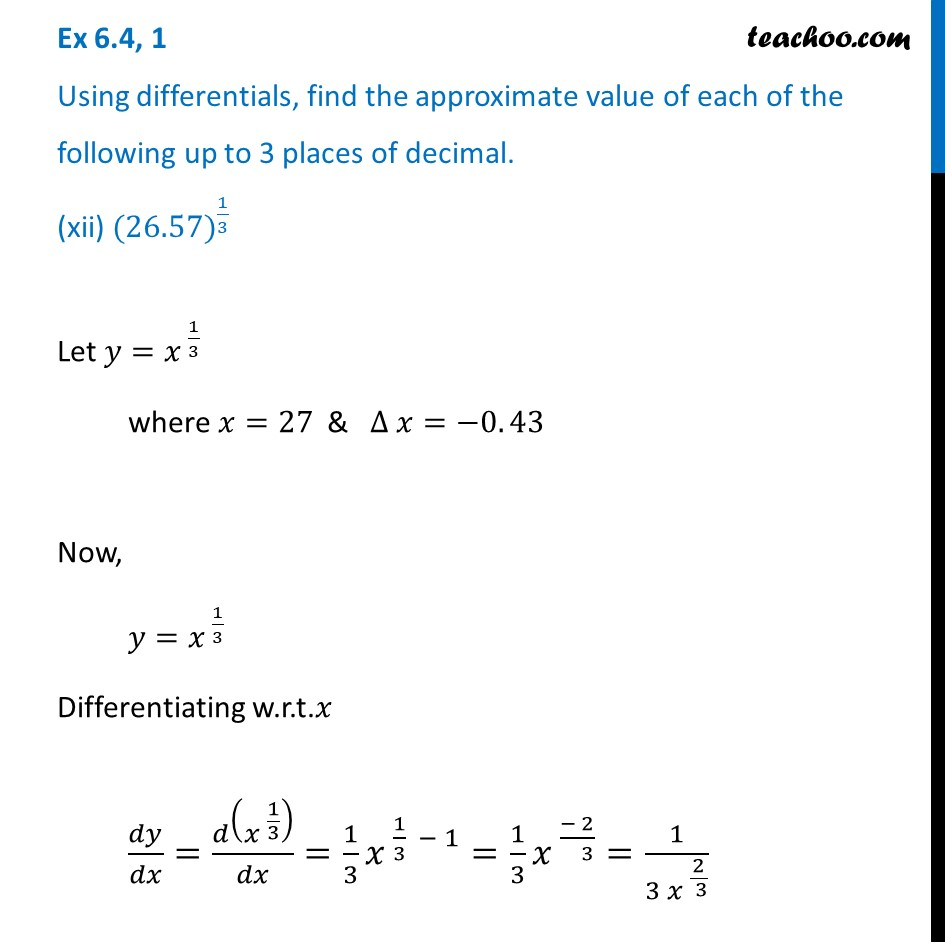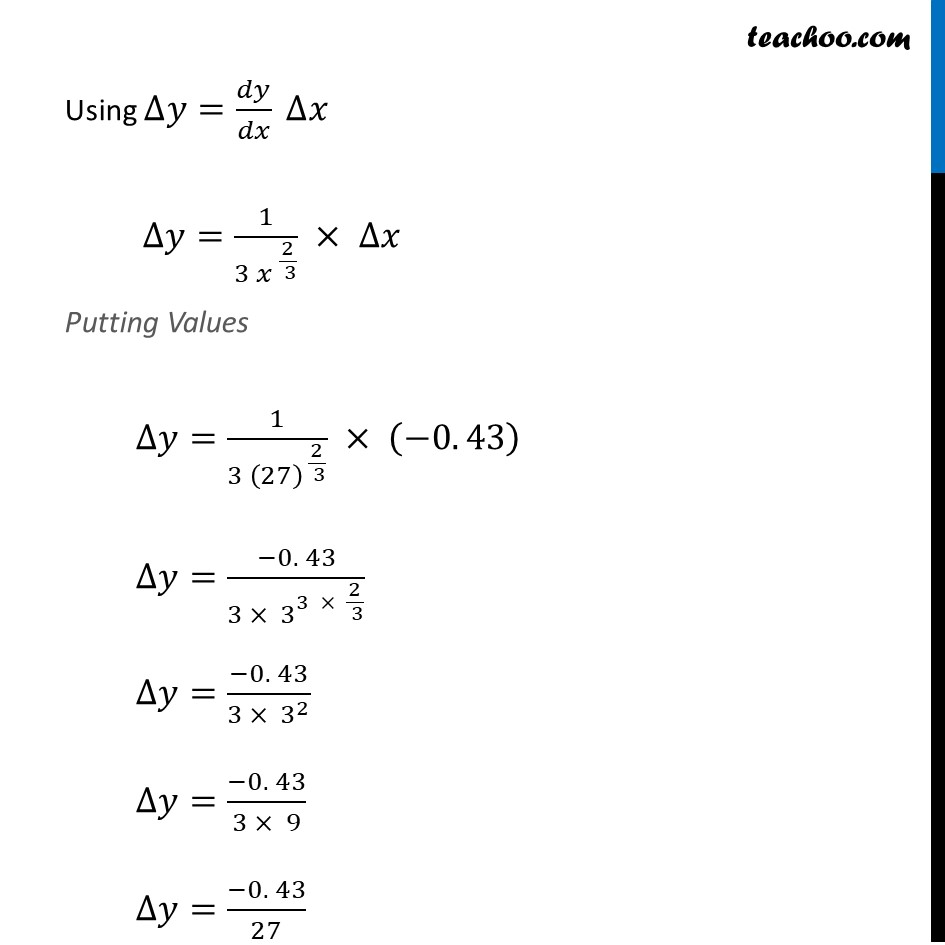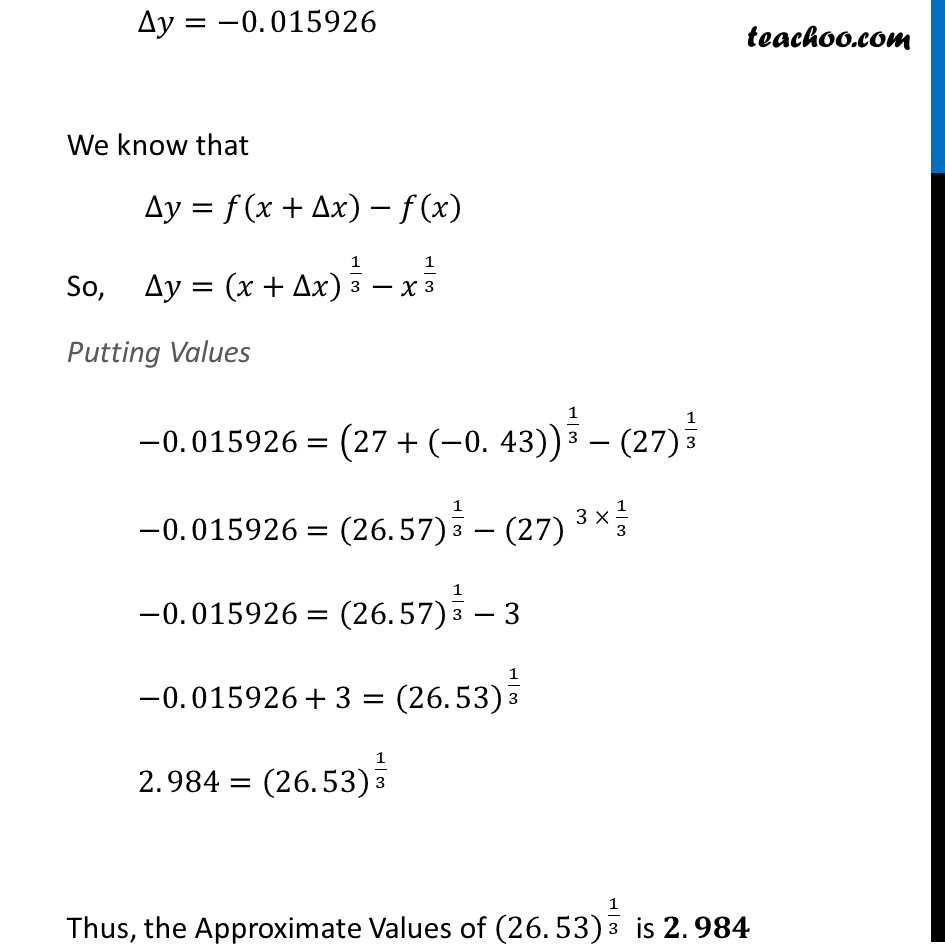Approximations (using Differentiation)

Chapter 6 Class 12 Application of Derivatives
Serial order wiseLearn in your speed, with individual attention - Teachoo Maths 1-on-1 Class

### Transcript

Question 1 Using differentials, find the approximate value of each of the following up to 3 places of decimal. (xii) 〖(26.57)〗^(1/3)Let 𝑦=𝑥^( 1/3) where 𝑥=27 & ∆ 𝑥=−0. 43 Now, 𝑦=𝑥^( 1/3) Differentiating w.r.t.𝑥 𝑑𝑦/𝑑𝑥=𝑑(𝑥^( 1/3) )/𝑑𝑥=1/3 𝑥^( 1/3 − 1)=1/3 𝑥^( (− 2)/( 3))=1/(3 𝑥^( 2/( 3)) ) Using ∆𝑦=𝑑𝑦/𝑑𝑥 ∆𝑥 ∆𝑦=1/(3 𝑥^( 2/( 3)) ) × ∆𝑥 Putting Values ∆𝑦=1/(3 (27)^( 2/( 3)) ) × (−0. 43) ∆𝑦=(−0. 43)/(3 × 3^(3 × 2/( 3)) ) ∆𝑦=(−0. 43)/(3 × 3^2 ) ∆𝑦=(−0. 43)/(3 × 9) ∆𝑦=(−0. 43)/27 ∆𝑦=−0. 015926 We know that ∆𝑦=𝑓(𝑥+∆𝑥)−𝑓(𝑥) So, ∆𝑦=〖(𝑥+∆𝑥) 〗^(1/3)−𝑥^( 1/3) Putting Values −0. 015926=(27+(−0. 43))^( 1/3)−(27)^( 1/3) −0. 015926=(26. 57)^( 1/3)−(27)^( 3 × 1/3) −0. 015926=(26. 57)^( 1/3)−3 −0. 015926+3=(26. 53)^( 1/3) 2. 984=(26. 53)^( 1/3) Thus, the Approximate Values of (26. 53)^( 1/3) is 𝟐. 𝟗𝟖𝟒